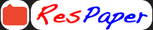Trending ▼   ResFinder# ICSE Class X Prelims 2021 : Mathematics (St. Johns Universal School, Mumbai)

3 pages, 20 questions, 10 questions with responses, 13 total responses,30Tanisha Thakur St. John's Universal School, Mumbai
+Fave Message
 Home > tanisha15102005 >   F Also featured on: School Page icse and 1 moreFormatting page ...

ST. JOHN S UNIVERSAL SCHOOL FORMATIVE ASSESSMENT 1 2020-2021 CLASS: X SUBJECT: MATHEMATICS MARKS: 50 TIME: 1 hr 50min. DATE: 17/08/2020 Answers to this paper must be written on a ruled sheet. First 10 minutes are to be spent in reading the question paper. The time given at the head of this paper is for writing and uploading the paper. The Answer Sheet should have the following details mentioned- Date, Full Name, Class & Division, Subject. The pages should be numbered appropriately. . Attempt all questions from section A and any THREE questions from section B. All working, including rough work must be clearly shown and must be done on the same sheet as the rest of the answer. Omission of essential working will result in loss of marks. The intended marks for questions or parts of questions are given in brackets [ ]. Mathematical tables are provided. Section A (20 marks) (Attempt all questions from this section) Question 1 a) Given x R, solve the inequation and graph the solution on the number line: 3 4 2 + 2 3 b) Two dice are rolled together. Find the probability of getting: i) A doublet ii) the product of the numbers as 4 or 5.   c) Ramesh has a recurring deposit account in a bank for three and a half years at 9.5 % p. a. If he deposits 1200 per month, find the interest paid by the bank and the maturity value of the account.  Question 2 a) Find the value of k for which the given equation has real and equal roots: 2 2( + 1) + 2 = 0  b) The marks scored by 16 students in a class test are as below: 3, 6, 8, 13, 15, 5, 21, 23, 17, 10, 9, 1, 20, 21, 18, 12. Find the median and mode.  c) Find vertex M of parallelogram LMNO, where L (10, -6), N (-4, -2) and O (4, -2).Formatting page ...

Top Contributors
to this ResPaperSneha Bansal(4)Sukrit Agarwal(2)Murugan S(2)Tom Mathew(2)Formatting page ...Additional Info : ICSE Class X Mid-term 2021 : Mathematics